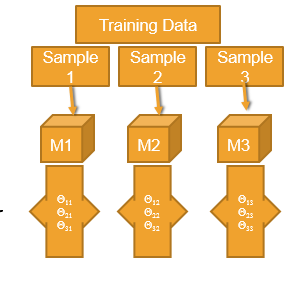• No products in the cart.

# 204.4.7 Problem of Overfitting

##### Understanding Over fitting with an example.
`Link to the previous post : https://statinfer.com/204-4-6-type-of-datasets-type-of-errors-and-problem-of-overfitting/`

### The Problem of Over Fitting

• In search of the best model on the given data we add many predictors, polynomial terms, Interaction terms, variable transformations, derived variables, indicator/dummy variables etc.,
• Most of the times we succeed in reducing the error. What error is this?
• So by complicating the model we fit the best model for the training data.
• Sometimes the error on the training data can reduce to near zero
• But the same best model on training data fails miserably on test data.
• Imagine building multiple models with small changes in training data. The resultant set of models will have huge variance in their parameter estimates.• The model is made really complicated, that it is very sensitive to minimal changes.
• By complicating the model the variance of the parameters estimates inflates.
• Model tries to fit the irrelevant characteristics in the data.
• Over fitting
• The model is super good on training data but not so good on test data
• We fit the model for the noise in the data
• Less training error, high testing error
• The model is over complicated with too many predictors
• Model need to be simplified
• A model with lot of variance

## Practice : Model with huge Variance

• Data: Fiberbits/Fiberbits.csv
• Take initial 90% of the data. Consider it as training data. Keep the final 10% of the records for validation.
• Build the best model(5% error) model on training data.
• Use the validation data to verify the error rate. Is the error rate on the training data and validation data same?
In :
```#Splitting the dataset into training and testing datasets
X = np.array(Fiber_df[features])
y = np.array(Fiber_df['active_cust'])

from sklearn.cross_validation import train_test_split
X_train, X_test, y_train, y_test = train_test_split(X,y, train_size = 0.9)
```
In :
```#Building model on training data.
tree_var = tree.DecisionTreeClassifier(criterion='gini',
splitter='best',
max_depth=20,
min_samples_split=2,
min_samples_leaf=1,
max_leaf_nodes=None)
tree_var.fit(X_train,y_train)
```
Out:
```DecisionTreeClassifier(class_weight=None, criterion='gini', max_depth=20,
max_features=None, max_leaf_nodes=None, min_samples_leaf=1,
min_samples_split=2, min_weight_fraction_leaf=0.0,
presort=False, random_state=None, splitter='best')```
In :
```#Accuracy of the model on training data
tree_var.score(X_train,y_train)
```
Out:
`0.95315555555555553`

Validation accuracy :

In :
```#Accuracy on the test data
tree_var.score(X_test,y_test)
```
Out:
`0.86550000000000005`
• Error rate on validation data is more than the training data error.

The next post is about  problem of under fitting.

Link to the next post : https://statinfer.com/204-4-8-problem-of-under-fitting/

21st June 2017
0 responses on "204.4.7 Problem of Overfitting"